# VDB Function

Get the depreciation of an asset using the Double Declining Balance method

## What is the VDB Declining Balance Function?

The VDB Function is an Excel Financial function that calculates the depreciation of an asset using the Double Declining Balance (DDB) method or some other method specified by the user. VDB is a short form of Variable Declining Balance.

The Excel VDB function also allows us to specify a factor to multiply the Straight-Line Depreciation by, although the function uses the DDB method by default. It will help a financial analyst in building financial models or creating a fixed asset depreciation schedule for analysis.

### Formula

=VDB(cost, salvage, life, start_period, end_period, [factor], [no_switch])

The VDB function uses the following arguments:

1. Cost (required argument) – This is the initial cost of the asset.
2. Salvage (required argument) – This is the value of an asset at the end of the depreciation. It can be zero. It is also known as the salvage value.
3. Life (required argument) – This is the useful life of the asset or the number of periods for which the asset will be depreciated.
4. Start_period (required argument) – The starting period for which you want to calculate the depreciation. Start_period must use the same units as life.
5. End_period (required argument) – This is the ending period for which you want to calculate the depreciation. End_period must use the same units as life.
6. Factor (optional argument) – This is the rate of depreciation. If we omit the argument, the function will take the default value of 2, which denotes the double declining balance method.
7. No_switch – This is an optional logical argument that specifies whether the method should switch to straight-line depreciation when depreciation is greater than the declining balance calculation. Possible values are:
1. TRUE – Excel will not switch to the straight-line depreciation method;
2. FALSE – Excel will switch to the straight-line depreciation method when depreciation is greater than the declining balance calculation.

### Calculate Declining Balance with the VDB Function in Excel

To understand the uses of the VDB function, let’s consider a few examples:

#### Example – Variable Declining Balance

Assume we wish to calculate the depreciation for an asset with an initial cost of \$500,000 and a salvage value of \$50,000 after 5 years.

We will calculate the depreciation for one day, one month, and one year. The formula used in calculating depreciation for a day is: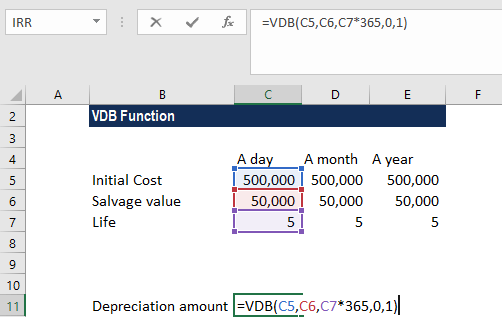We get the result below: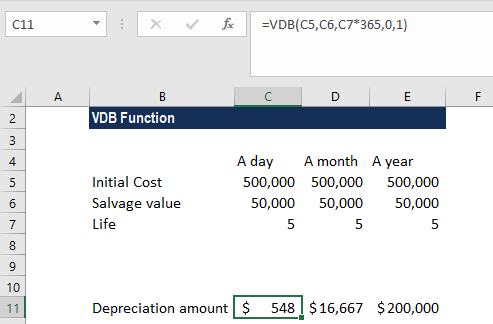The formula used in calculating depreciation for a month is: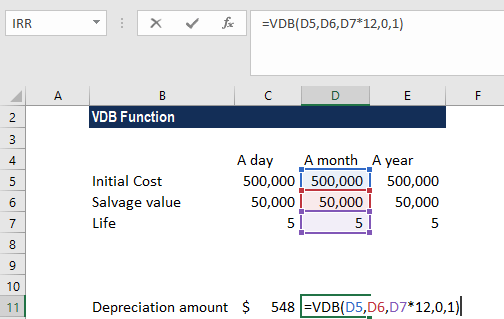We get the result below: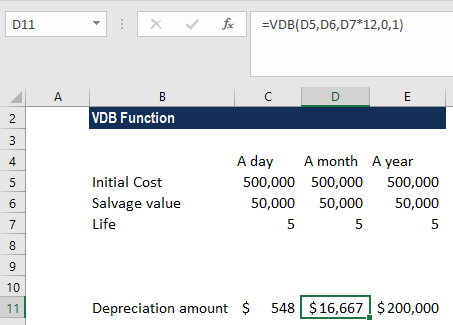The formula used in calculating depreciation for a year is: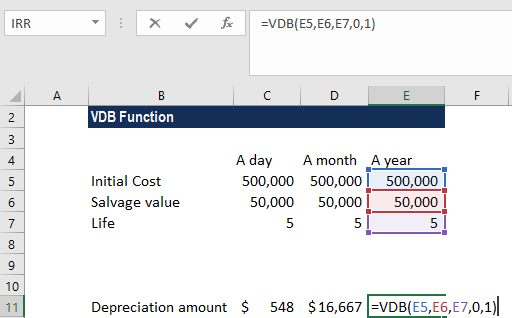We get the result below: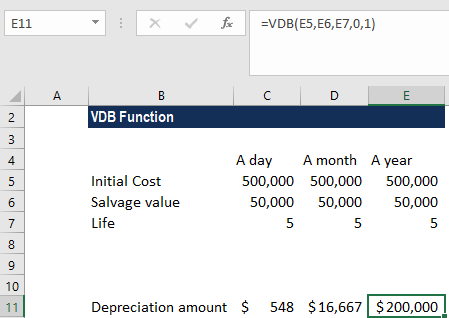In the formulas above, we did not provide the factor argument, so Excel assumed it as 2 and used the DDB method. Excel also assumed the no_switch argument as FALSE.

### Things to remember about the VDB Function

1. We need to provide arguments “period” and “life” in the same units of time: years, months, or days.
2. All arguments except no_switch must be positive numbers.
3. #VALUE! error – Occurs when the given arguments are non-numeric.
4. #NUM! error – Occurs when:
• Any of the supplied cost, salvage, start_period, end_period or [factor] arguments are < 0;
• The given life argument is less than or equal to zero;
• The given start_period is > the given end_period; and
• Start_period > life or end_period > life.

Thanks for reading CFI’s guide to the Excel VDB function. By taking the time to learn and master these functions, you’ll significantly speed up your financial analysis. To learn more, check out these additional CFI resources:

• Excel Functions for Finance